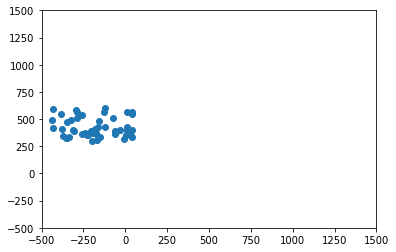Close

MPHY0021: Research Software Engineering With Python

Home## Exercise: Refactoring The Bad Boids¶

We have written some very bad code implementing our Boids flocking example.

git clone      git@github.com:yourname/bad-boids.git


For the Exercise, you should start from the GitHub repository, but here's our terrible code:

In :
"""
[Boids](http://dl.acm.org/citation.cfm?doid=37401.37406)
for use as an exercise on refactoring.
"""

from matplotlib import pyplot as plt
from matplotlib import animation

import random

# Deliberately terrible code for teaching purposes

boids_x=[random.uniform(-450,50.0) for x in range(50)]
boids_y=[random.uniform(300.0,600.0) for x in range(50)]
boid_x_velocities=[random.uniform(0,10.0) for x in range(50)]
boid_y_velocities=[random.uniform(-20.0,20.0) for x in range(50)]
boids=(boids_x,boids_y,boid_x_velocities,boid_y_velocities)

def update_boids(boids):
xs,ys,xvs,yvs=boids
# Fly towards the middle
for i in range(len(xs)):
for j in range(len(xs)):
xvs[i]=xvs[i]+(xs[j]-xs[i])*0.01/len(xs)
for i in range(len(xs)):
for j in range(len(xs)):
yvs[i]=yvs[i]+(ys[j]-ys[i])*0.01/len(xs)
# Fly away from nearby boids
for i in range(len(xs)):
for j in range(len(xs)):
if (xs[j]-xs[i])**2 + (ys[j]-ys[i])**2 < 100:
xvs[i]=xvs[i]+(xs[i]-xs[j])
yvs[i]=yvs[i]+(ys[i]-ys[j])
# Try to match speed with nearby boids
for i in range(len(xs)):
for j in range(len(xs)):
if (xs[j]-xs[i])**2 + (ys[j]-ys[i])**2 < 10000:
xvs[i]=xvs[i]+(xvs[j]-xvs[i])*0.125/len(xs)
yvs[i]=yvs[i]+(yvs[j]-yvs[i])*0.125/len(xs)
# Move according to velocities
for i in range(len(xs)):
xs[i]=xs[i]+xvs[i]
ys[i]=ys[i]+yvs[i]

figure=plt.figure()
axes=plt.axes(xlim=(-500,1500), ylim=(-500,1500))
scatter=axes.scatter(boids,boids)

def animate(frame):
update_boids(boids)
scatter.set_offsets(list(zip(boids,boids)))

anim = animation.FuncAnimation(figure, animate,
frames=200, interval=50)If you go into your folder and run the code:

cd bad_boids
python boids.py


You should be able to see some birds flying around, and then disappearing as they leave the window.

In :
from IPython.display import HTML
HTML(anim.to_jshtml())

Out: